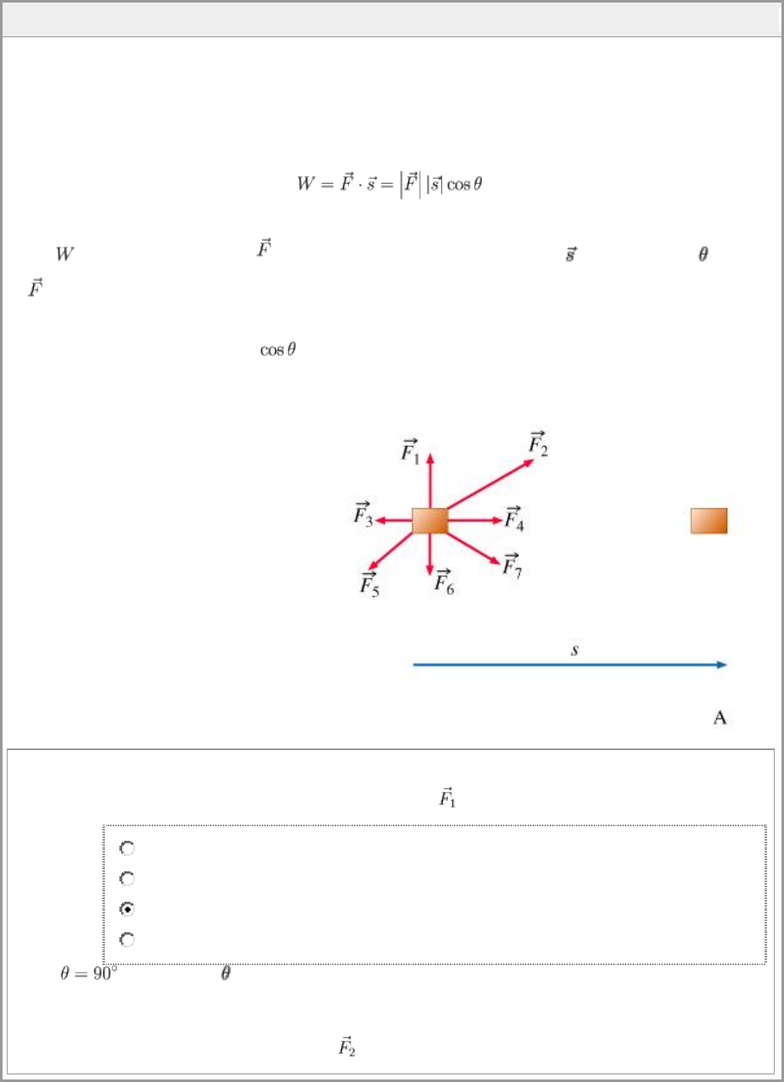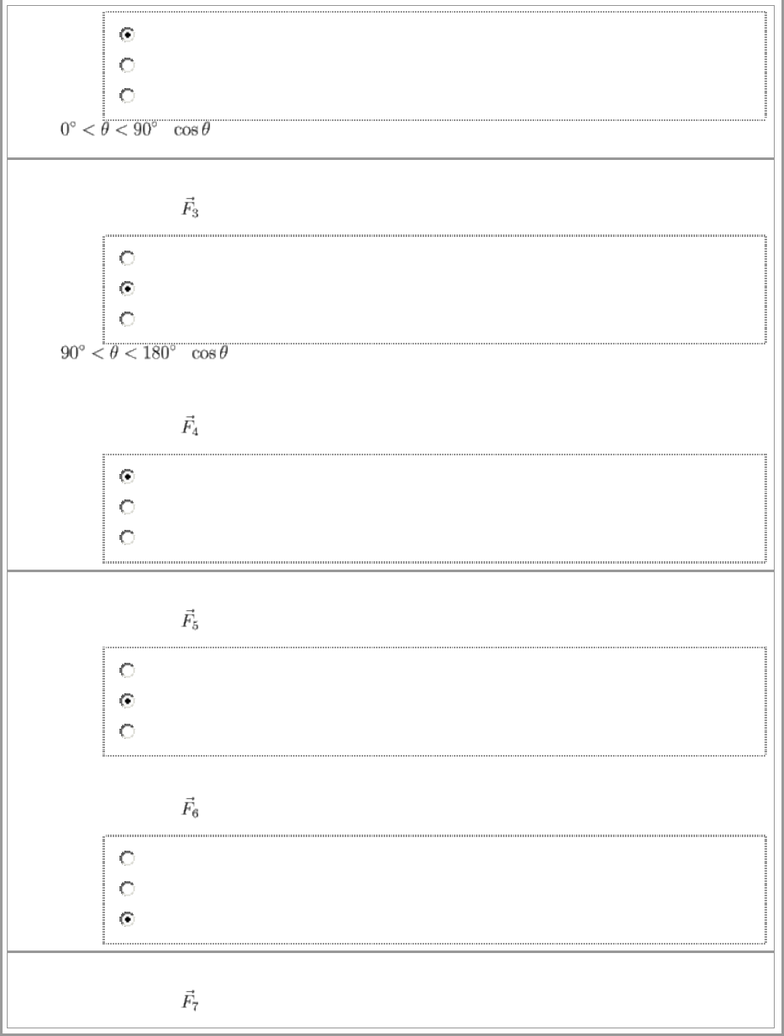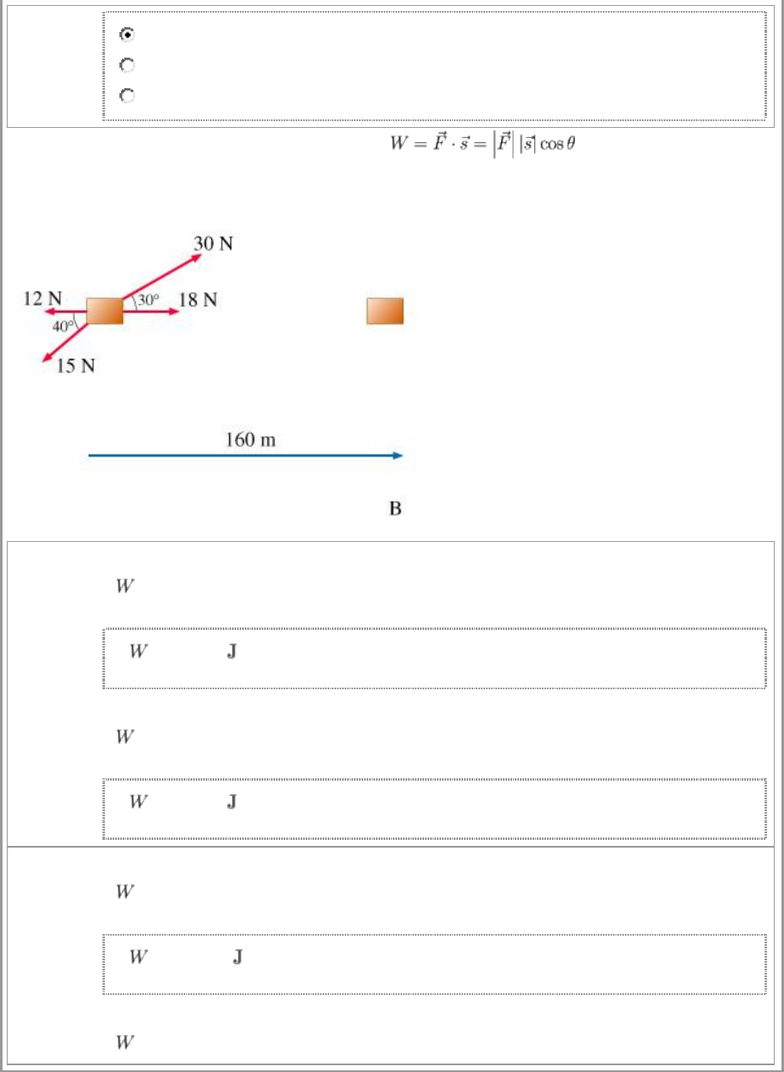Study Guides (400,000)
US (230,000)
ASU (1,000)
PHY (10)
All (7)

# homework 6 mastering physics solution university physics

Department
Physics
Course Code
PHY 121
Professor
All

This preview shows pages 1-3. to view the full 20 pages of the document.All Work and No Play
Learning Goal: To be able to calculate work done by a constant force directed at different angles relative to
displacement
If an object undergoes displacement while being acted upon by a force (or several forces), it is said that work is
being done on the object. If the object is moving in a straight line and the displacement and the force are known,
the work done by the force can be calculated as
,
where is the work done by force on the object that undergoes displacement directed at angle relative
to .
Note that depending on the value of , the work done can be positive, negative, or zero.
In this problem, you will practice calculating work done on an object moving in a straight line. The first series of
questions is related to the accompanying figure.
Part A
What can be said about the sign of the work done by the force ?
It is positive.
It is negative.
It is zero.
There is not enough information to answer the question.
When , the cosine of is zero, and therefore the work done is zero.
Part B
What can be said about the work done by force ?

Only pages 1-3 are available for preview. Some parts have been intentionally blurred.It is positive.
It is negative.
It is zero.
When , is positive, and so the work done is positive.
Part C
The work done by force is
positive
negative
zero
When , is negative, and so the work done is negative.
Part D
The work done by force is
positive
negative
zero
Part E
The work done by force is
positive
negative
zero
Part F
The work done by force is
positive
negative
zero
Part G
The work done by force is

Only pages 1-3 are available for preview. Some parts have been intentionally blurred.positive
negative
zero
In the next series of questions, you will use the formula
to calculate the work done by various forces on an object that moves 160 meters to the right.
Part H
Find the work done by the 18-newton force.
=
2900
Part I
Find the work done by the 30-newton force.
=
4200
Part J
Find the work done by the 12-newton force.